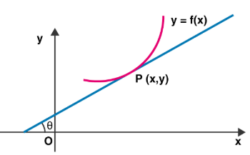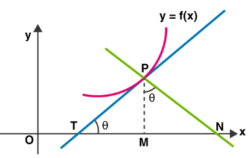# Subtangent And Subnormal

A line can be called a tangent to the differentiable curve, y = f(x) at a point P if it makes an angle Θ at the x-axis. The derivative dy / dx = tan Θ is the slope of the tangent to the curve at a point.Equation of normal at (x1, y1) is $y-y_{1} = \frac{-1}{\frac{dy}{dx}} (x-x_{1})$

where the slope is calculated at the point (x, y).

## Few Important Points

• The slope of the tangent of the curve is given by the derivative of the curve equation with respect to x.
• The slope of the normal is given by $\frac{-1}{\frac{dy}{dx}} (x_1, y_1)$.
• The tangent equation is given by (y – b) = $\frac{-dx}{dy} (x-a)$.
• If x2 + y2 + 2gx + 2fy + c = 0 is a circle and a point is taken outside the circle such that a line drawn from the point to proceed through the boundary of a circle by coming in contact with the tangency point is given by, $y-(-f)=m(x-[-g]*\sqrt{1+m^2})$

## Subtangent And Subnormal Definition

Let y = f (x) be the curve that is differentiable at a point P. Let the tangent and normal at P(x, y) to the curve meet at the x-axis at points T and N. M is the projection of P on the x-axis. In the figure below,

• PT is the length of the tangent
• PN is the length of the normal
• TM is the length of subtangent
• MN is the length of the subnormal## Subtangent And Subnormal Formulae

The formula for subtangent and subnormal is given below.

• The length of the tangent PT = $PT=|y|\sqrt{1+[\frac{dx}{dy}]^2}$
• The length of the subtangent PM = $TM=|ycot\Theta |=|y*\frac{dx}{dy}|$
• The length of the normal PN = $PN=|y|\sqrt{1+[\frac{dy}{dx}]^2}$
• The length of the subnormal MN = $TM=|ycot\Theta |=|y*\frac{dy}{dx}|$

## Subtangent And Subnormal Solved Examples

Example 1: What is the equation of the tangent to the curve y = be[−x/a] at the point where it crosses the y-axis?

Solution:

y = be[−x/a] meets the y-axis at (0, b).

Again, y = be[−x/a] (−1 / a)

At (0, b), dy / dx = be0 (−1 / a) = −b / a

Therefore, the required tangent is y − b = −b / a (x−0) or x / a + y / b = 1

Example 2: If x + 4y = 14 is normal to the curve y2 = ax3 − β at (2, 3), then find the value of α+β.

Solution:

y2 = ax3 − β or dy / dx = 3αx2 / 2y

Therefore, slope of the normal at (2, 3) is (−dx / dy) (2,3) = −2 × 3 / 3α (2)2 = −12α = −14 Or α = 2 Also, (2, 3) lies on the curve.

Therefore, 9 = 8α − β or β = 16 − 9 = 7

or

α+β = 9

Example 3: If the normal to the curve is 2x2 + y2 = 12 at the point (2, 2) then at which of the following point does the curve cuts the normal again?

A) (−22 / 9, −29)

B) (22 / 9, 2 / 9)

C) (−2,−2)

D) None of these

Solution:

2x2 + y2 = 12 or dy / dx = −2x / y.

The slope of normal at point A (2, 2) is 1 / 2.

Also, point B (−22 / 9,−2 / 9) lies on the curve and slope of AB is

[2−(−2/9)] / [2−(−22/9)] = 1 / 2

Hence, the normal meets the curve again at point (−22 / 9,−2 / 9).

Example 4: The lines tangent to the curves y3 − x2 y + 5y − 2x = 0 and x4 − x3y2 + 5x + 2y = 0 at the origin intersect at an angle θ equal to ______.

Solution:

Differentiating y3 − x2 y + 5y − 2x = 0 w.r.t. x,

we get 3 y2 y′− 2xy − x2y′ + 5y′ − 2 = 0

Or y′ = [2xy + 2] / [3y2 − x2 +5]

or y′(0,0) = 2 / 5

Differentiating x4 − x3y2 + 5x + 2y = 0 w.r.t. x,

we get 4x2 − 3x2y2 − 2x3yy′ + 5 + 2y′= 0

Or y′= [3x2y2 −4x3 − 5] / [2 − 2x3y]

or y′(0,0) = −5 / 2

Thus, both the curves intersect at a right angle.

Example 5: If the length of subnormal is equal to the length of subtangent at a point (3, 4) on the curve y = f (x) and the tangent at (3, 4) to y = f (x) meets the coordinate axes at A and B, then calculate the maximum area of the triangle OAB, where O is origin.

Solution:

Length of subnormal = length of the subtangent

or dy/ d x = ±1

If dy / dx = 1, equation of the tangent is y − 4 = x − 3 or y − x = 1

The area of ΔOAB = [1 / 2] × 1 × 1 = 1 / 2

If dy / dx = −1, the equation of the tangent is y − 4 = −x + 3 Or y + x = 7,

Area = [1 / 2] × 7 × 7 = 49 / 2

Example 6: For the curve $b{{y}^{2}}={{(x+a)}^{3}}$ the square of subtangent is proportional to

$A) {{\text{(Subnormal)}}^{1/2}}\\ B) \text \ Subnormal\\ C) {{\text{(Subnormal)}}^{\text{3/2}}}\\ D) \text \ None \ of \ these$

Solution:

$b{{y}^{2}}={{(x+a)}^{3}}\\ \Rightarrow 2by.\frac{dy}{dx}=3{{(x+a)}^{2}}\Rightarrow \frac{dy}{dx}=\frac{3}{2by}{{(x+a)}^{2}} \\ \text \ Subnormal = y\frac{dy}{dx}=\frac{3}{2b}{{(x+a)}^{2}} \\ \text \ Subtangent = \frac{y}{\left( \frac{dy}{dx} \right)}=\frac{y}{\frac{3{{(x+a)}^{2}}}{2by}}=\frac{2b{{y}^{2}}}{3{{(x+a)}^{2}}} = \frac{2b{{\frac{(x+a)}{b}}^{3}}}{3{{(x+a)}^{2}}}=\frac{2}{3}(x+a) \\ \text \ (Subtangent)^{2} = \frac{4}{9}{{(x+a)}^{2}} \text \ and \frac{{{(\text{Subtangent})}^{2}}}{\text{Subnormal}}=\frac{\frac{4}{9}{{(x+a)}^{2}}}{\frac{3}{2b}{{(x+a)}^{2}}}=\frac{8b}{27} \\(\text \ Subtangent)^{2} = \text \ constant ´ (\text \ Subnormal).$

Example 7: If ST and SN are the lengths of the subtangent and the subnormal at the point $\theta =\frac{\pi }{2}$ on the curve $x=a(\theta +\sin \theta ),y=a(1-\cos \theta ),a\ne 1$, then

$A) ST=SN\\ B) ST=2\,SN\\ C) S{{T}^{2}}=a\,S{{N}^{3}}\\ D) S{{T}^{3}}=a\,SN\\$

Solution:

$\frac{dx}{d\theta }=a(1+\cos \theta ),\,\frac{dy}{d\theta }=a\,(\sin \theta ) \\ {{\left. \frac{dy}{dx} \right|}_{\theta =\frac{\pi }{2}}}=\frac{\frac{dy}{d\theta }}{\frac{dx}{d\theta }}=\frac{a\sin \theta }{a(1+\cos \theta )}=1,\\ {{\left. y \right|}_{\theta =\frac{\pi }{2}}}=a$

Length of sub-tangent ST = $\frac{y}{dy/dx}=\frac{a}{1}=a.$

Length of the sub-normal SN = $y\frac{dy}{dx}=a\,.\,1=a$

Hence ST = SN.

Example 8: For the curve $xy={{c}^{2}}$ the subnormal at any point varies as

$A) {{x}^{2}}\\ B) {{x}^{3}}\\ C) {{y}^{2}}\\ D) {{y}^{3}}$

Solution:

$xy={{c}^{2}}\rightarrow (i)\text \ because \ Subnormal \ = y\frac{dy}{dx} \\ \text \ From \ (i), \ y=\frac{{{c}^{2}}}{x}\\ \frac{dy}{dx}=\frac{-{{c}^{2}}}{{{x}^{2}}} \\ \text \ Subnormal \ =\frac{y\times (-{{c}^{2}})}{{{x}^{2}}}=\frac{-y{{c}^{2}}}{{{\left( \frac{{{c}^{2}}}{y} \right)}^{2}}}=\frac{-y{{c}^{2}}{{y}^{2}}}{{{c}^{4}}}=\frac{-{{y}^{3}}}{{{c}^{2}}} \\ \text \ Subnormal \ varies \ as \ {{y}^{3}}.$

Example 9: If the curve $y={{a}^{x}}$ and $y={{b}^{x}}$ intersect at angle $\alpha$, then find $\tan \alpha$.

Solution:

Clearly the point of intersection of curves is (0, 1).

Now, the slope of the tangent of the first curve

${{m}_{1}}=\frac{dy}{dx}={{a}^{x}}\log a\\{{\left( \frac{dy}{dx} \right)}_{(0,\,1)}}={{m}_{1}}=\log a$

Slope of tangent of second curve

${{m}_{2}}=\frac{dy}{dx}={{b}^{x}}\log b \\ {{m}_{2}}={{\left( \frac{dy}{dx} \right)}_{(0,\,1)}}=\log b \\ \tan \alpha =\frac{{{m}_{1}}-{{m}_{2}}}{1+{{m}_{1}}{{m}_{2}}}\\=\frac{\log a-\log b}{1+\log a\log b}.$